# AP Chemistry : Calorimetry, Specific Heat, and Calculations

## Example Questions

### Example Question #1 : Calorimetry, Specific Heat, And Calculations

The following is a list of specific heat capacities for a few metals.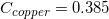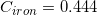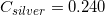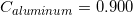A 50g sample of an unknown metal is heated with 800 joules. If the temperature of the metal increases by 41.6oC, what is the identity of the unknown metal?

Aluminum

Silver

Copper

Iron

Copper

Explanation:

We need to find the specific heat of the unknown sample of metal in order to locate it on the list. We can do this by using the equation that allows us to determine the specific heat capacity of an element.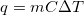Since we know the change in temperature, we can simply plug in the values and solve for the value of.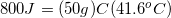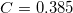Going back to the list, we see that this is the specific heat capacity for copper, so we confirm that the unknown metal is copper.

### Example Question #1 : Calorimetry, Specific Heat, And Calculations

In which instance would a bomb calorimeter be more useful than a coffee-cup calorimeter?

When measuring the mass of a substance

None of the above

When measuring the specific heat of water vapor

When measuring the heat capacity of a solid

When measuring the specific heat of water vapor

Explanation:

Bomb calorimeters are most useful when dealing with a gas, because they can operate well at high pressures. Coffee-cup calorimeters are not useful when water begins to boil, producing vapor.

### Example Question #1 : Calorimetry, Specific Heat, And Calculations

How much heat does it take to heat 100g ice at 0C to boiling point?

Cice= 2.1 J/goC

Cwater= 4.2 J/goC

ΔHvap= 2260 J/g

ΔHfus=334 J/g

33.4 kJ

75.4 kJ

42 kJ

55.4 kJ

300.5 kJ

75.4 kJ

Explanation:

You need heat for the phase change, using the enthalpy of fusion (100g*334 J/g = 33400 J). Add to this the heat to get to boiling point using the specific heat of water (100g*100C*4.2 J/goC = 42000 J). Totalling 75400 J (75.4 kJ)

### Example Question #4 : Calorimetry, Specific Heat, And Calculations

The specific heat capacity of an unknown liquid is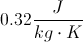. The density of the liquid is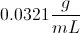If a chemist applies 243 J of heat to 300 mL of this liquid starting at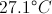, what is the final temperature?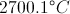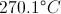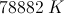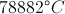Explanation:

First we will determine the mass of the liquid: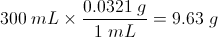Now we will examine the relationship between heat and specific heat capacity: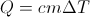Where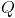is heat in Joules,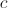is the specific heat capacity,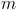is the mass and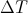is the change in temperature. We can rearrange this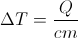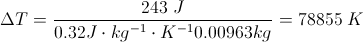If we begin at, we will end at### Example Question #1 : Calorimetry, Specific Heat, And Calculations

A 50g sample of a metal was heated to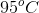then quickly transferred to an insulated container containing 50g of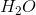at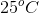.  The final temperature of thewas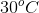.

Which of the following can be concluded?

The specific heat of the metal is greater than that of the water

The metal lost an amount of thermal energy that was more than the amount of thermal energy gained by the water

The specific heat of the water is greater than that of the metal

The water gained an amount of thermal energy that was more than the amount of thermal energy lost by the metal

The specific heat of the water is greater than that of the metal

Explanation:

When the heated metal is placed in the container of the cooler water there will be a transfer of thermal energy from the metal to the water.  This transfer will occur towards an equilibrium of thermal energy in the water and in the metal. Thus we can conclude that the amount of thermal energy lost by the metal will equal the amount of thermal energy gained by the water.  However we notice that the water increases by only 5oC  and the metal decreases by 65oC.  This is becasue of the difference of the specific heats of these substances.  The specific heat capacity of a substance is the heat required to increase the temperature of 1g of a substance by 1oC. The metal can be conluded to have a smaller specific heat than the water because the same amount of energy transfer led to a much larger change in termperature for the metal as compared to the water.

### Example Question #1 : Calorimetry, Specific Heat, And Calculations

Which of the following is the correct molar specific heat of water used when making calculations involving a calorimeter?

0.4184 J/gK

4.184 J/gK

41.84 J/gK

418.4 J/gK

4.184 J/gK

Explanation:

4.184 J/gK is the cited value for the specific heat of water and should be memorized. This is used during calorimeter calculations, specifically when using the equation q= mc delta(T).

### Example Question #7 : Calorimetry, Specific Heat, And Calculations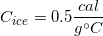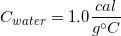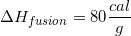How much energy is needed to raise the temperature of five grams of ice from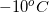to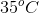?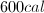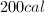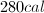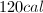Explanation:

This question involves the total energy needed for three different processes: the temperature raise fromto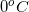, the melting of the ice, and the temperature raise fromto. For the first and third transitions we will use the equation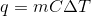. For the melting of ice, we will use the equation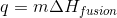.

1.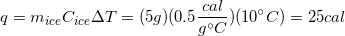2.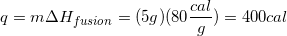3.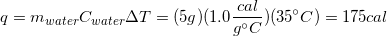Finally, we will need to sum the energy required for each step to find the total energy.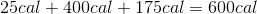### Example Question #1 : Calorimetry, Specific Heat, And Calculations

You want to prepare a cup of tea. To do so, you pour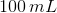of tap water at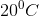in a cup that does not absorb microwave radiation and heat it in a microwave oven at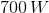of power. If you assume a density of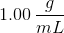for the water and know that its specific heat capacity is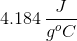, what time do you need to set in the microwave oven to heat the water to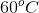?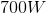of power is not enough to heat that a amount of water to the desired temperature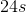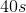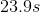Explanation: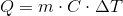Since the density of water is, the mass ofis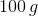. Plug in known values to the equation and solve.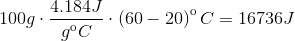Use the formula below to find the time needed to heat up the sample of water in the microwave: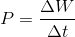Our answer must contain three significant figures.

### Example Question #1 : Calorimetry, Specific Heat, And Calculations

How much heat is needed to raisegrams of aluminum by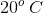?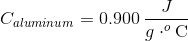.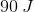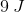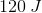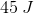Explanation:

To find the amount of heat needed to change the temperature of a given material by a certain amount, we'll need to use the equation for specific heat. The specific heat capacity of a compound represents the amount of energy necessary to raisegram of that substance by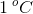.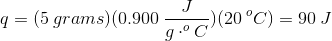### Example Question #1 : Thermodynamics

A 20g sample of iron at a temperature of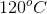is placed into a container of water. There are 300 milliliters of water in the container at a temperature of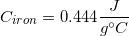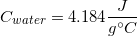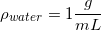What is the final temperature of the water?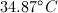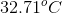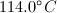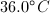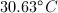Explanation:

There are two things to note before solving for the final temperature.

1. The density of water allows us to say that 300 milliliters of water is the same thing as 300 grams of water.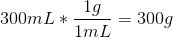2. Since the heat from the iron is being transferred to the water, we can say that the heat transfer is equal between both compounds. Since the heat is conserved in the system, we can set the two equations equal to one another.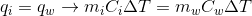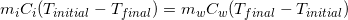Notice how the change in temperature for iron has been flipped in order to avoid a negative number.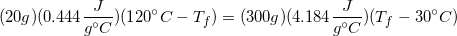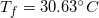Because water has a much higher heat capacity compared to iron, the temperature of the water is not changed significantly.

### All AP Chemistry Resources